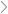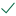Questions & AnswersManagerial AccountingQuestion 13 Given a risk-adjusted discount rate of 14%, calculate...

QuestionAnswered step-by-step

# Question 13 Given a risk-adjusted discount rate of 14%, calculate...

Question 13

Given a risk-adjusted discount rate of 14%, calculate the discounted payback period for the following cash flow of a project. If the maximum target discounted payback period is 3 years, comment on the financial acceptability of the project.(a)   Accept the project, as the target payback period is 3, which is shorter than the adjusted payback period of 2.32.

(b)   Turn down the project, as the adjusted payback period is 4.23, which is longer than the target period of 3 years.

(c)    Turn down the project, as the target payback period is 3, which is shorter than the adjusted payback period of 3.79.

(d)   Accept the project, as the adjusted payback period is 1.79, which is shorter than the target period of 3 years.Solved by verified expert
<p><strong>sectetur adipiscing elit. N</strong>                                     </p>sectetur adipiscing elit. Nam lacinia pulvinar tortor nec facilisis. Pellentesque dapibus efficitur laoreet. Nam risus ante, dapibus a molestie consequat, ultrices ac magna. Fusce dui lectus, congue vel laoreet ac, dictum v10,000 step-by-step explanations